# Modeling Notes

2015/09/16 05:36

### 1. Stats

Standard deviation - how spread out the data is

s = sqrt[ sum of power(Xi - Xmean, 2) / (n-1)], n-1 for sample, n for entire population

Variance - square of SD

Covariance - measure between 2 dimension, vary from the mean with respect to each other

s = sqrt[ sum of (Xi - Xmean) * (Yi - Ymean) / (n-1)]

Covariance matrix

Eigenvectors - all the eigenvectors of a matrix are orthogonal

Eigenvalues eigenvectors and eigenvalues always come in pairs

solve for A -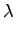I

A -I =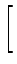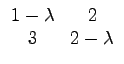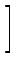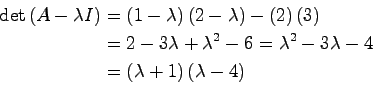### 2. Goodness of fit

Estimate model parameters

1. Maximum likelihood estimation method (MLE)

2. Least squares estimation method (LSE)

1. find the parameter values that make the observed data most likely

Accessing model fitness

The total sum of squares (proportional to the variance of the data):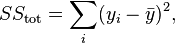The regression sum of squares, also called the explained sum of squares: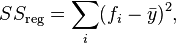The sum of squares of residuals, also called the residual sum of squares: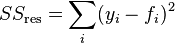R-squared =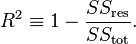= Explained variation / Total variation, which is always between 0 and 100%

AIC and BIC

Pearson correlation coefficient =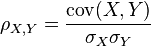= Covariance / SDx*SDy

the two variables in question must be continuous, not categorical

Chi-square test

is used to show whether or not there is a relationship between two categorical variables

Likelihood ratio test

have been used to compare two models

T-test

is used to test whether there is a difference between two groups on a continuous dependent variable

ANOVA

is very similar to the t-test, but it is used to test differences between three or more groups

Significance Level (Alpha)

is the probability of rejecting the null hypothesis when it is true

P-value

The p-value for each term tests the null hypothesis that the coefficient is equal to zero

P-values are the probability of obtaining an effect at least as extreme as the one in your sample data, assuming the truth of the null hypothesis.

Regression coefficients

represent the mean change in the response variable for one unit of change in the predictor variable while holding other predictors in the model constant

Linear vs nonlinear model

A model is linear when each term is either a constant or the product of a parameter and a predictor variable. A linear equation is constructed by adding the results for each term. This constrains the equation to just one basic form: Response = constant + parameter * predictor + ... + parameter * predictor

Y = b o + b1X1 + b2X2 + ... + bkXk

Reference :

How Do I Interpret R-squared and Assess the Goodness-of-Fit

How to Interpret a Regression Model with Low R-squared and Low P values

Use Adjusted R-Squared and Predicted R-Squared to Include the Correct Number of Variables

R-squared Shrinkage and Power and Sample Size Guidelines for Regression Analysis

### 3. Categorical variable

Dummy Coding

Effect Coding

Orthogonal Coding

Criterion Coding

Lasso

Group Lasso

Modified Group Lasso

### 4. Random Forest

Algorithm

1. Draw ntree bootstrap samples from the original data.

2. For each of the bootstrap samples, grow an unpruned classification or regression tree, with the following modification: at each node, rather than choosing the best split among all predictors, randomly sample mtry of the predictors and choose the best split from among those variables. (Bagging can be thought of as the special case of random forests obtained when mtry = p, the number of predictors.)

3. Predict new data by aggregating the predictions of the ntree trees (i.e., majority votes for classification, average for regression).

An estimate of the error rate can be obtained, based on the training data, by the following:

1. At each bootstrap iteration, predict the data not in the bootstrap sample (what Breiman calls “out-of-bag”, or OOB, data) using the tree grown with the bootstrap sample.

2. Aggregate the OOB predictions. (On the average, each data point would be out-of-bag around 36% of the times, so aggregate these predictions.) Calcuate the error rate, and call it the OOB estimate of error rate.

The randomForest package optionally produces two additional pieces of information: a measure of the importance of the predictor variables, and a measure of the internal structure of the data (the proximity of different data points to one another).

Variable importance This is a difficult concept to define in general, because the importance of a variable may be due to its (possibly complex) interaction with other variables. The random forest algorithm estimates the importance of a variable by looking at how much prediction error increases when (OOB) data for that variable is permuted while all others are left unchanged. The necessary calculations are carried out tree by tree as the random forest is constructed. (There are actually four different measures of variable importance implemented in the classification code. The reader is referred to Breiman (2002) for their definitions.)

Proximity measure The (i, j) element of the proximity matrix produced by randomForest is the fraction of trees in which elements i and j fall in the same terminal node. The intuition is that “similar” observations should be in the same terminal nodes more often than dissimilar ones. The proximity matrix can be used

### 6. Rank Deficiency

Rank deficiency in this context says there is insufficient information contained in your data to estimate the model you desire. It stems from many origins.

a. Too little data. Replication only helps to reduce noice, but not Rank deficiency. As replication of two points still give you a straight line, not quardratic model.

b. Wrong data pattern. You cannot fit a two dimensional quadratic model if all you have are points that all lie in a straight line in two dimensions.

c. Units and scaling. The mathematics will fail when a computer program tries to add and subtract numbers that vary by so many orders of magnitude.

0
0 收藏

### 作者的其它热门文章

0 评论
0 收藏
0